# The area of triangle whose adjacent sides are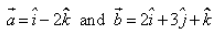is :a)√70/2 sq. unitsb)9√2 /2 sq. unitsc)3√3 /2 sq. unitsd)2√3 /2 sq. unitsCorrect answer is option 'A'. Can you explain this answer? Related Test: Test: Cross Product

## JEE QuestionShivani Jun 12, 2018Suresh Kumar May 13, 2020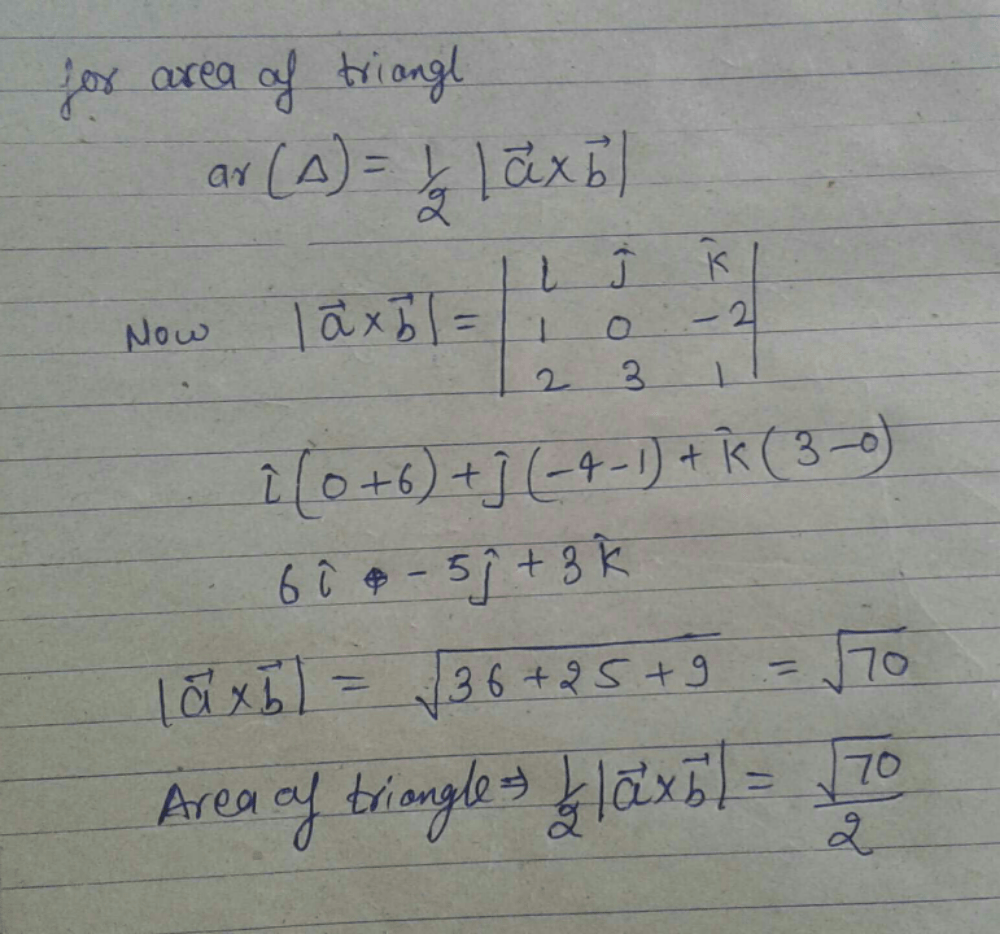Vikas Singh Nov 17, 2020
A cross B by 2 is the answer will give the area of triangle because A cross B gives area of parallelogram and in parallelogram it is divided into 2 equal parts so divide the area of parallelogram by 2 gives the area of triangleAishwary Omar Apr 21, 2020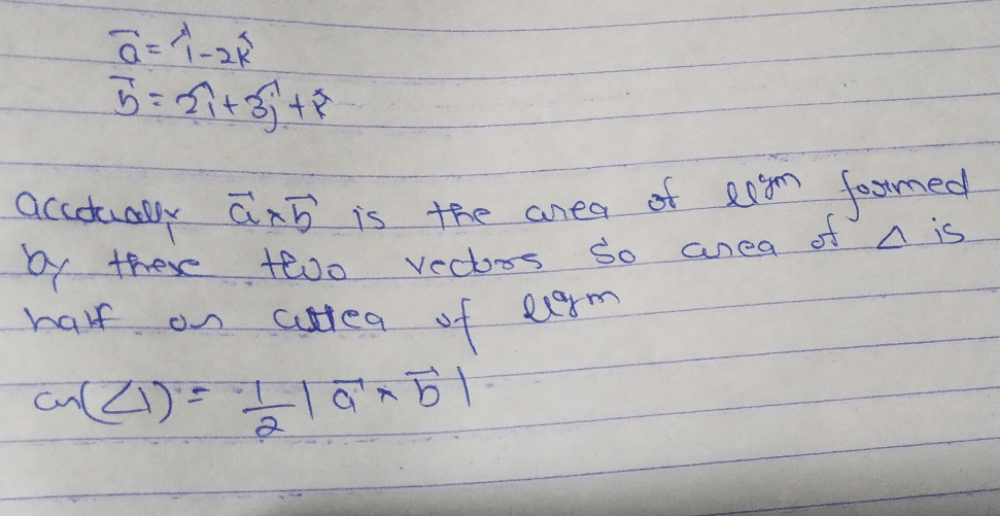Asiya Anjum Jun 01, 2020
￼Asiya anjumNisarga P7 4 days ago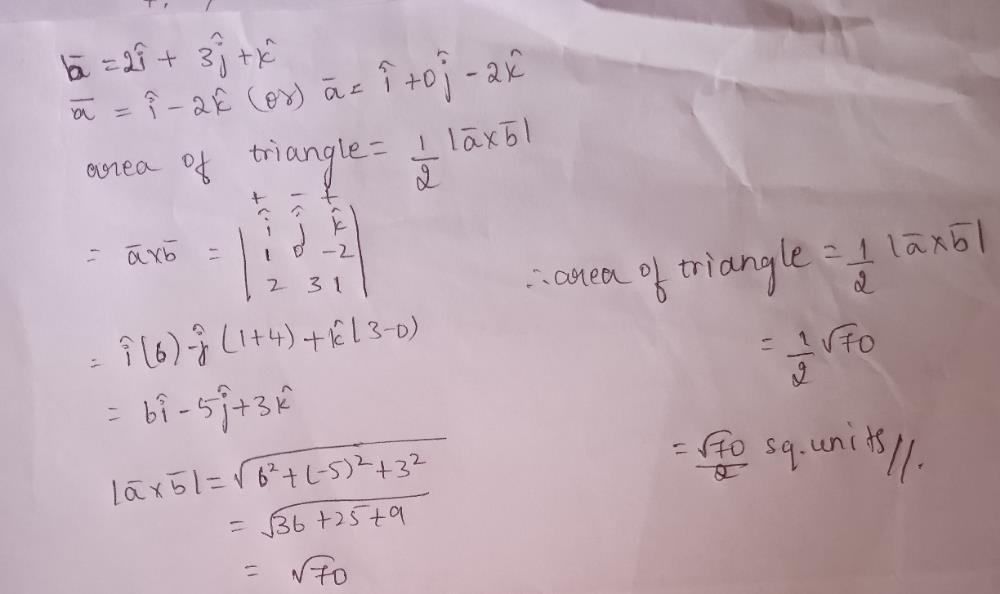Shashmith Shashmith Mar 24, 2020
Area = 1/2 × | a × b | = √70/2Navya Hegde Apr 09, 2020
Hence option A is the correct oneUppuluri Sv Subbarao May 02, 2020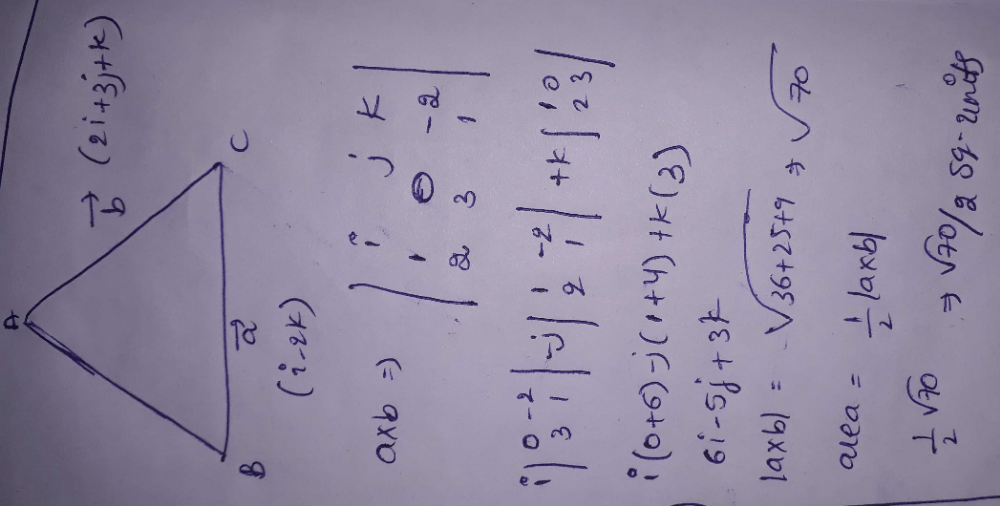Mohit Mittal Jul 01, 2020
Area = 1/2 ( cross product of both vectors of adjacent side )Gurram Samanidhar Reddy Mar 28, 2020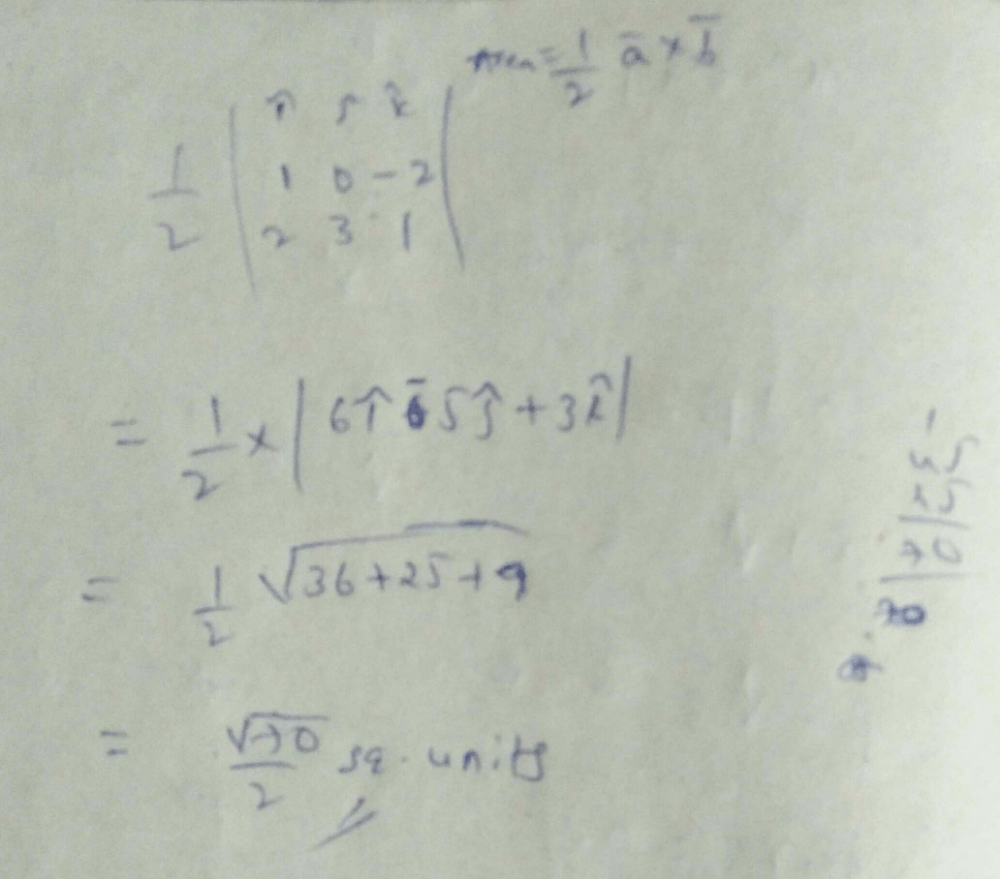Ankurkumar Ankurkumar Apr 23, 2020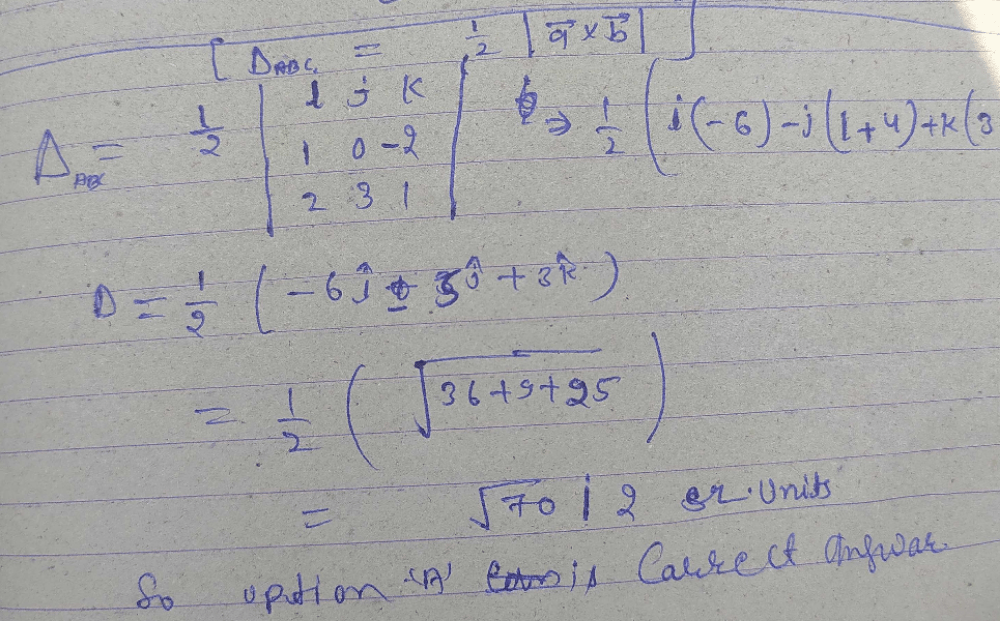Srija Rachakonda May 29, 2020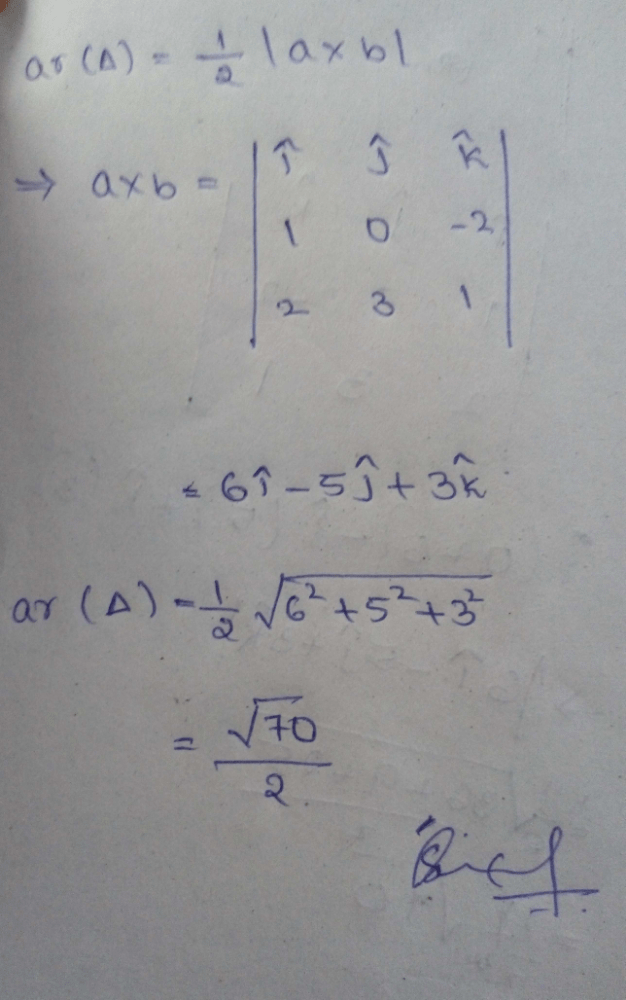Mani Chari May 28, 2020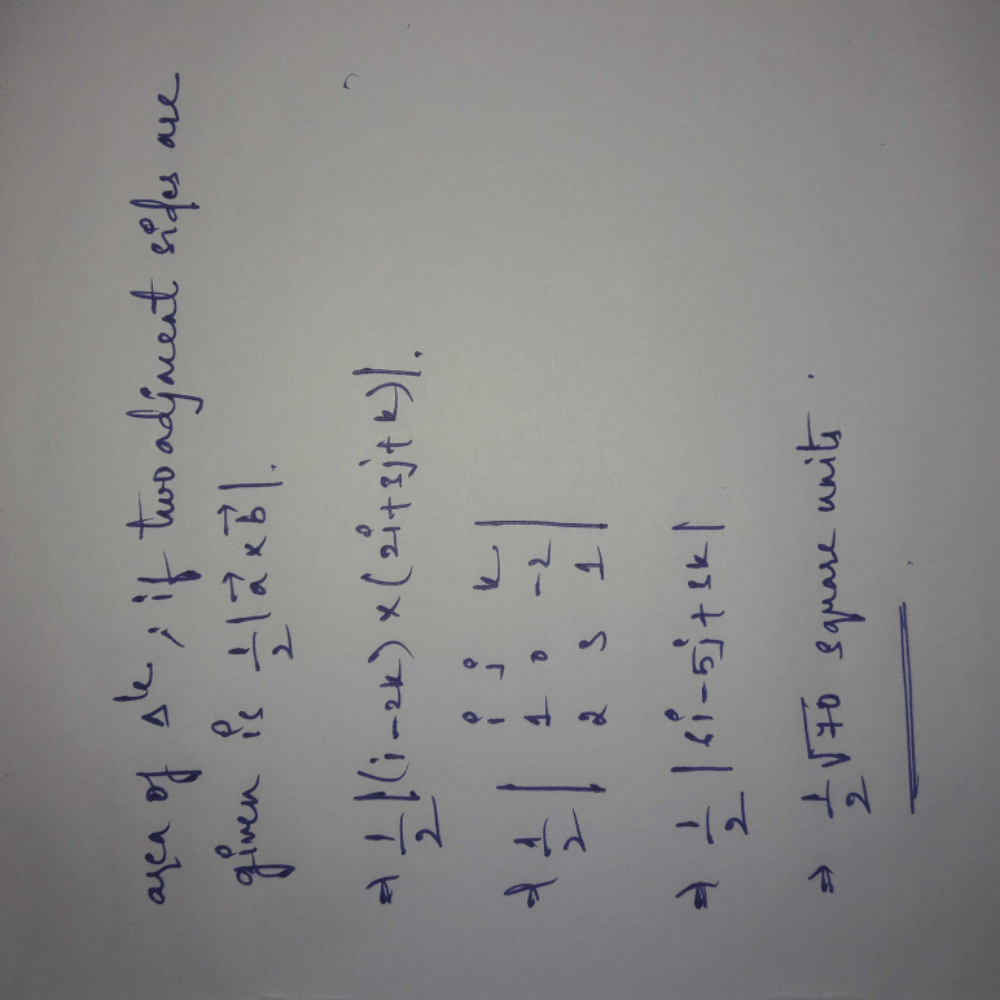Md Altamash Alam Apr 15, 2020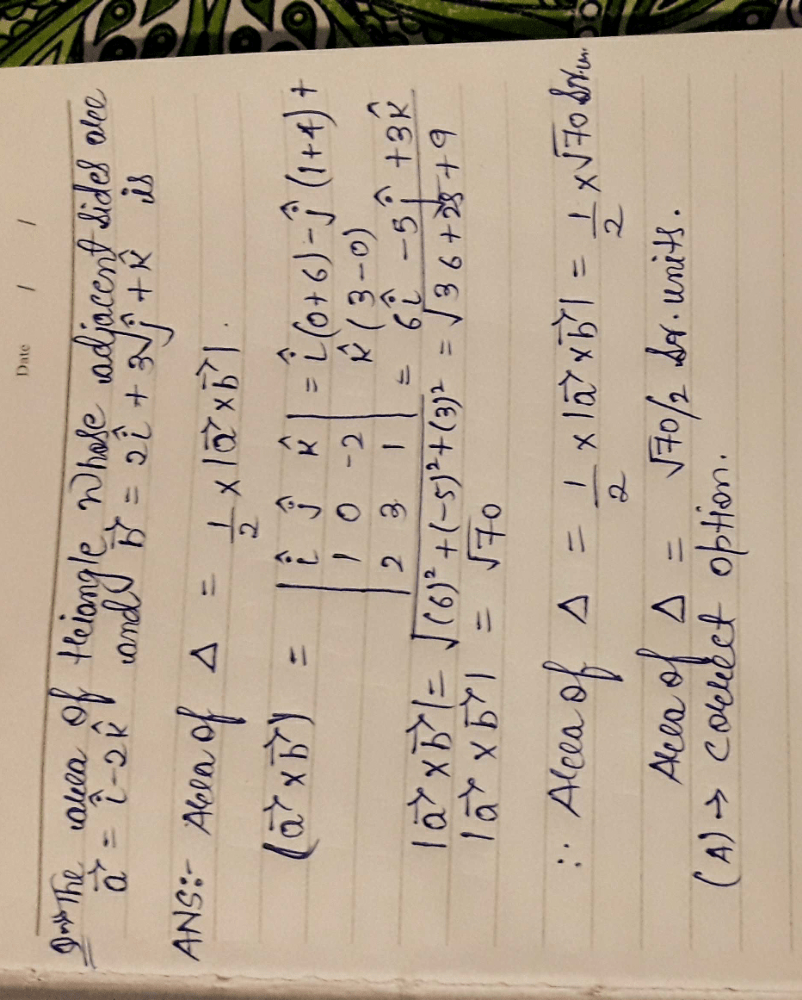Shaurya Sawai Sep 16, 2020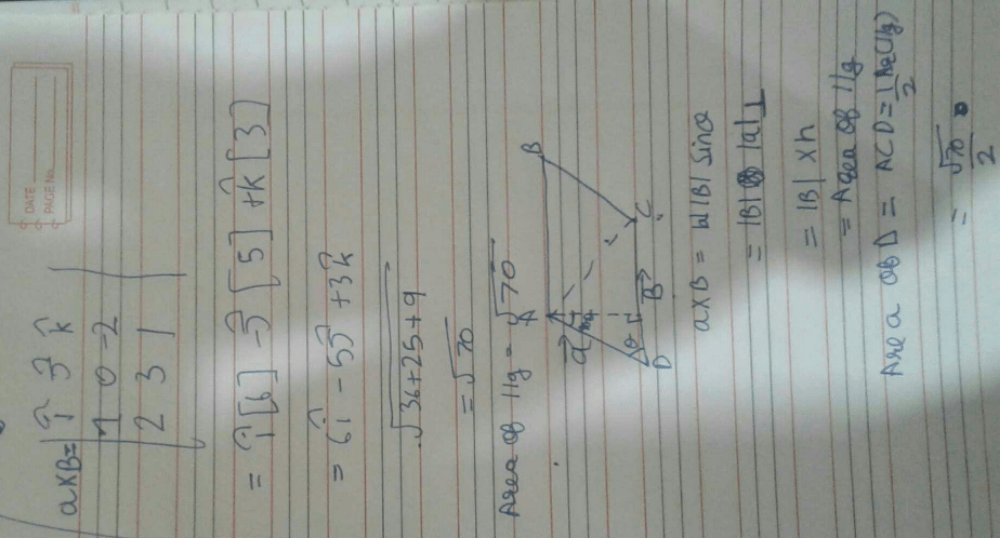Shubham Karande Aug 29, 2018
Do the cross product. You will get the area of parallelogram and then divide it by 2, you will get the area of triangleShweta Bhamu May 28, 2020
Area of triangle =1/2×modulous (axb) wher 'a' and 'b' adjacents side vecto of triangle.Shreya Singh Sep 16, 2018
Area of triangle is =1/2|ab|.
on doing ab cross product =|6i^-5j^+3k^|=√36+25+9=√70...
area of triangle=1/2(√70).
=√70/2....Aman Kumar Jul 21, 2020
Correct option aAman Choudhary Jul 21, 2020
A hogaHareesh Kumar Jul 12, 2020
ASudesh Kumar Jun 07, 2020
Cross multiply the two vectors by matrices or by other methods and then divide by 2Gayatri Devi Aug 19, 2020
You will easily get the answer by the standard cross product formulae :(|a×b|)/2.sqrt70/2 is the answer.Krit Goswami Jul 01, 2020